# Version information¶

:

%matplotlib notebook
from PySide2.QtWidgets import *
from datetime import date
print("Running date:", date.today().strftime("%B %d, %Y"))
import pyleecan
print("Pyleecan version:" + pyleecan.__version__)
import SciDataTool
print("SciDataTool version:" + SciDataTool.__version__)

Running date: May 20, 2022
Pyleecan version:1.4.0
SciDataTool version:2.4.0


# How to plot results¶

Pyleecan has generic built-in plot methods which allow to easily plot:

• simulated or experimental fields (unit conversions supported)

• slices (normalizations available)

• Fourier Transforms

but also to compare on the same graph a list of fields.

This flexibility is provided by the SciDataTool module, which has been created to ease the handling of scientific data, and considerately simplify plot commands. It unifies the extraction of relevant data (e.g. slices), whether they are stored in the time/space or in the frequency domain. The call to Fourier Transform functions is transparent, although it still can be parameterized.

This tutorial explains how to use Pyleecan’s plot commands. It is available on GitHub. For more details on the SciDataTool package, please refer to the specific tutorials:

To demonstrate the capabilities and the use of the SciDataTool objects, two simulations are launched with FEMM: with imposed currents and in open-circuit. The simulations use periodicity and parallelization to reduce execution time.

:

# Import Pyleecan modules
from numpy import exp, sqrt, pi
from os.path import join
from pyleecan.Classes.Simu1 import Simu1
from pyleecan.Classes.InputCurrent import InputCurrent
from pyleecan.Classes.OPdq import OPdq
from pyleecan.Classes.MagFEMM import MagFEMM
from pyleecan.Classes.ForceMT import ForceMT
from pyleecan.Classes.Output import Output
from pyleecan.definitions import DATA_DIR

# Simulation initialization
simu = Simu1(name="FEMM_periodicity", machine=Toyota_Prius)

# Definition of the enforced output of the electrical module
simu.input = InputCurrent(
Na_tot=252 * 8,
Nt_tot=50 * 8,
)
# Set Id/Iq according to I0/Phi0
simu.input.OP = OPdq(N0=1000)
simu.input.OP.set_I0_Phi0(I0=250 / sqrt(2), Phi0=140*pi/180)

# Definition of the magnetic simulation: with periodicity
simu.mag = MagFEMM(is_periodicity_a=True, is_periodicity_t=True, nb_worker=4)
simu.force = ForceMT(is_periodicity_a=True, is_periodicity_t=True)

# Definition of the open-circuit simulation
simu2 = simu.copy()
simu2.input.OP.set_Id_Iq(Id=0,Iq=0)

# Run simulations
out = simu.run()
out2 = simu2.run()

[17:41:10] Starting running simulation FEMM_periodicity (machine=Toyota_Prius)
[17:41:10] Starting Magnetic module
[17:41:12] Solving time steps: 0%
[17:41:12] Solving time steps: 0%
[17:41:12] Solving time steps: 0%
[17:41:13] Solving time steps: 0%
[17:41:17] Solving time steps: 25%
[17:41:17] Solving time steps: 25%
[17:41:18] Solving time steps: 25%
[17:41:19] Solving time steps: 25%
[17:41:22] Solving time steps: 50%
[17:41:22] Solving time steps: 50%
[17:41:23] Solving time steps: 50%
[17:41:23] Solving time steps: 50%
[17:41:27] Solving time steps: 75%
[17:41:28] Solving time steps: 75%
[17:41:28] Solving time steps: 75%
[17:41:30] Solving time steps: 75%
[17:41:32] Solving time step: 100%
[17:41:32] Solving time step: 100%
[17:41:32] Solving time step: 100%
[17:41:34] Solving time step: 100%
[17:41:34] Starting Force module
[17:41:34] End of simulation FEMM_periodicity
[17:41:34] Starting running simulation FEMM_periodicity (machine=Toyota_Prius)
[17:41:34] Starting Magnetic module
[17:41:35] Solving time steps: 0%
[17:41:35] Solving time steps: 0%
[17:41:36] Solving time steps: 0%
[17:41:37] Solving time steps: 0%
[17:41:45] Solving time steps: 25%
[17:41:45] Solving time steps: 25%
[17:41:49] Solving time steps: 25%
[17:41:50] Solving time steps: 25%
[17:41:52] Solving time steps: 50%
[17:41:53] Solving time steps: 50%
[17:41:57] Solving time steps: 50%
[17:41:58] Solving time steps: 50%
[17:42:03] Solving time steps: 75%
[17:42:04] Solving time steps: 75%
[17:42:04] Solving time steps: 75%
[17:42:05] Solving time steps: 75%
[17:42:08] Solving time step: 100%
[17:42:09] Solving time step: 100%
[17:42:09] Solving time step: 100%
[17:42:10] Solving time step: 100%
[17:42:10] Starting Force module
[17:42:10] End of simulation FEMM_periodicity


The following example demonstrates the syntax to quickly plot the torque and airgap flux obtained at the end of the simulation:

:

%matplotlib notebook
#------------------------------------------------------
# Plot the torque as a function of time
out.mag.Tem.plot_2D_Data("time")
out.mag.B.plot_2D_Data("angle")
#------------------------------------------------------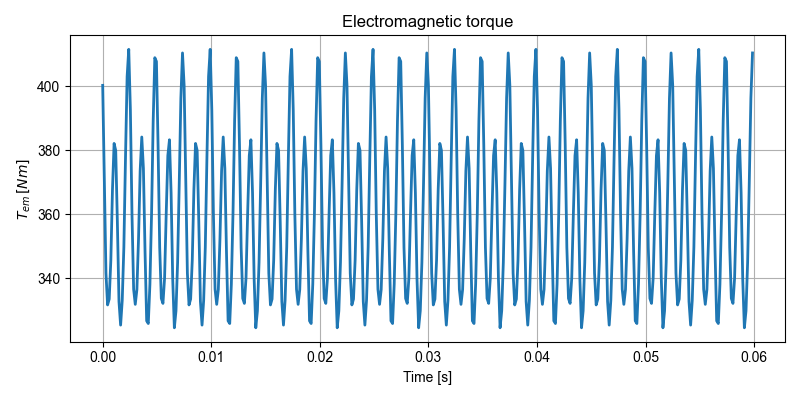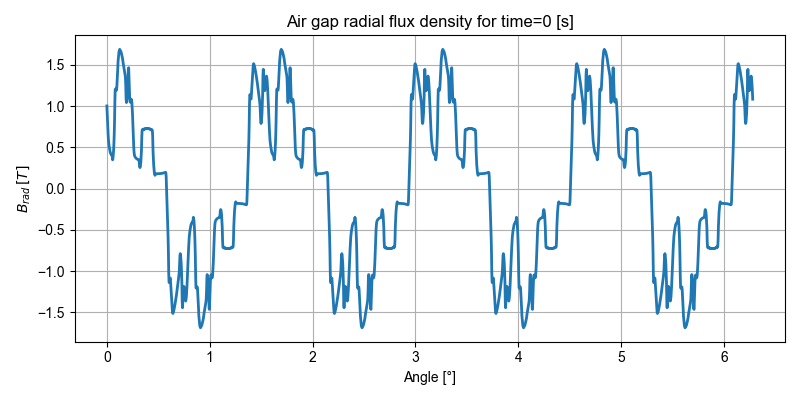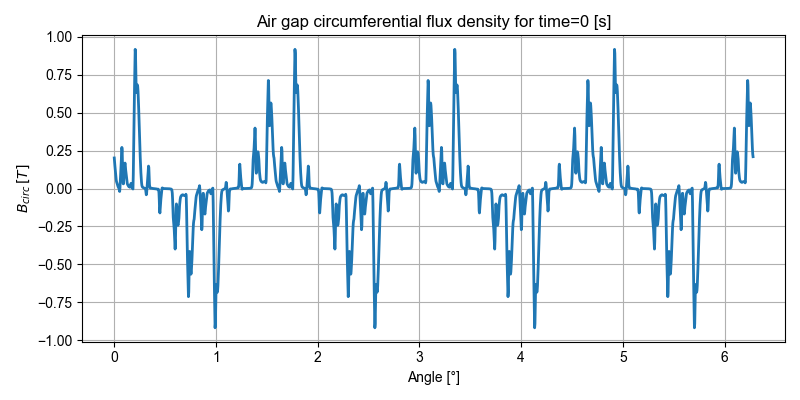The plot command requires the axis of the field along which to plot (“angle” for instance). Titles and legends are automatically generated. The commands work with any field computed in Pyleecan (and stored in a SciDataTool object).

The syntax for the axes is specific to SciDataTool objects, and will be detailed in this tutorial.

There are two main plot commands in SciDataTool, which can apply to any DataTime, DataFreq or VectorField object from SciDataTool:

• plot_2D_Data: field as a function of one dimension (time, angle, freqs, wavenumber, phase

• plot_3D_Data: field as a function of two dimensions

SciDataTool also provides generic plot functions, which can be useful to easily format other plots, or plots of data which is not stored in a SciDataTool object:

• plot_2D: plot as a function of one dimension (curve, bargraph, barchart, quiver, curve_point, point, barStackResultant)

• plot_3D: plot as a function of two dimensions (stem, surf, pcolor, scatter)

• plot_4D: plot as a function of three dimensions (scatter)

To use one of these plot functions, simply import it such as:

:

from SciDataTool.Functions.Plot.plot_2D import plot_2D


We invite you to visit SciDataTool repository on GitHub for more details.

Fonts and colors can be automatically defined from the config_dict, using dict_2D or dict_3D:

:

from pyleecan.Functions.Plot import dict_2D, dict_3D


## 1. How to plot only one period/antiperiod¶

To plot only one period or anti-period:

:

#---------------------------------------------------------------
# Plot the torque along the smallest period of time
out.mag.Tem.plot_2D_Data("time[smallestperiod]", **dict_2D)
#---------------------------------------------------------------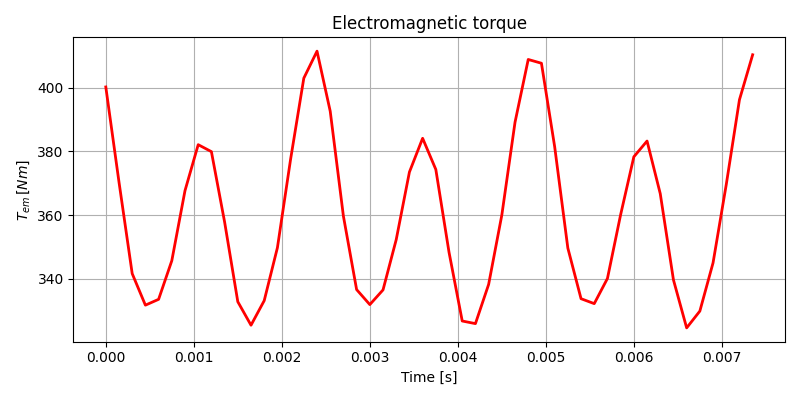## 2. How to plot VectorField objects¶

For VectorField objects, such as mag.B or force.AGSF, a plot for each component will be created:

:

#---------------------------------------------------------------
# Plot all components of flux density
out.mag.B.plot_2D_Data("time", **dict_2D)
#---------------------------------------------------------------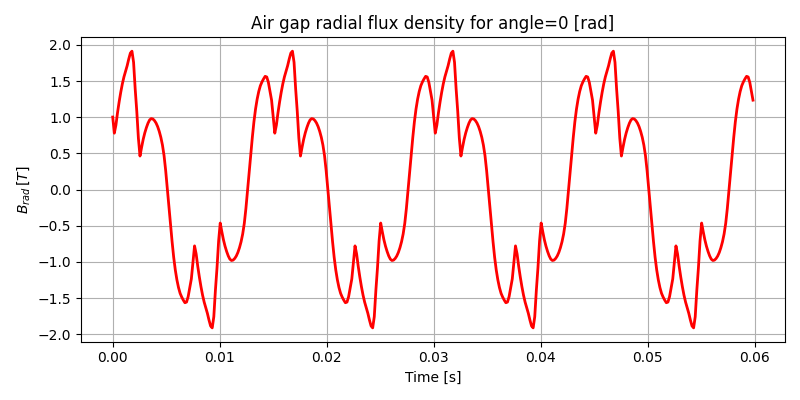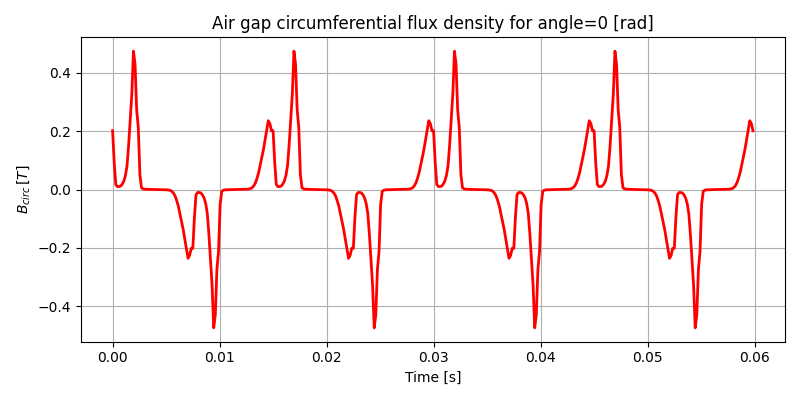To plot only one or several specific components, one can use the component_list parameter:

:

#---------------------------------------------------------------
# Plot only radial flux density